﻿ Natural convection in a rectangular region with radiation - XSim

# Natural convection in a rectangular region with radiation

Update: June 1, 2017
OpenFOAM 4.x

## Summary

We calculate the steady flow around a heating body (region "box") with a temperature of 500 K placed in a rectangular region. The bottom (region "floor") and the top (region "ceilling") are assumed to have a temperature of 300 K. The sides (region "fixedWalls") are assumed to be thermally opened to allow heat to escape. And no fluid flow into or out of the analysis domain.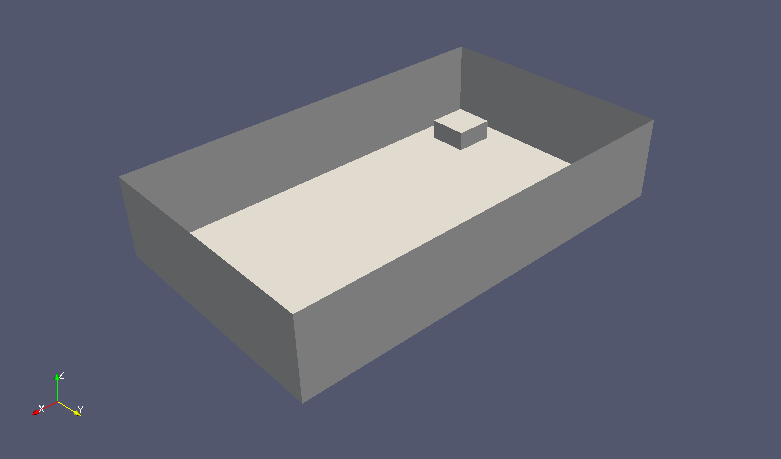Model geometry

We consider radiation's effect in the analysis. The radiation settings is specified in the file constant/radiationProperties as follows.

```radiation on;
solverFreq 1;

absorptionEmissionModel constantAbsorptionEmission;
constantAbsorptionEmissionCoeffs
{
absorptivity    absorptivity    [0 -1 0 0 0 0 0] 0.5;
emissivity      emissivity      [0 -1 0 0 0 0 0] 0.5;
E               E               [1 -1 -3 0 0 0 0] 0;
}

scatterModel    none;
sootModel       none;
```

The magnitude and direction of the gravity acceleration is specified in the file constant/g as follows, and the direction is the negative direction of the Z-axis.

```dimensions      [0 1 -2 0 0 0 0];
value           (0 0 -9.81);
```

The meshes are as follows, and the number of mesh is 104000.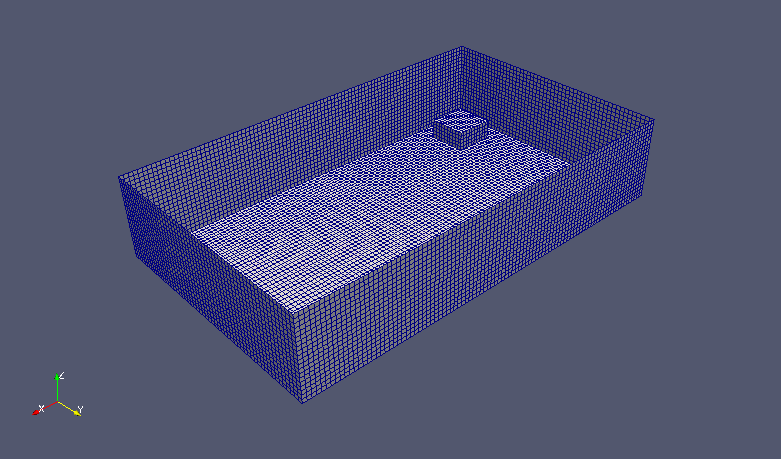Meshes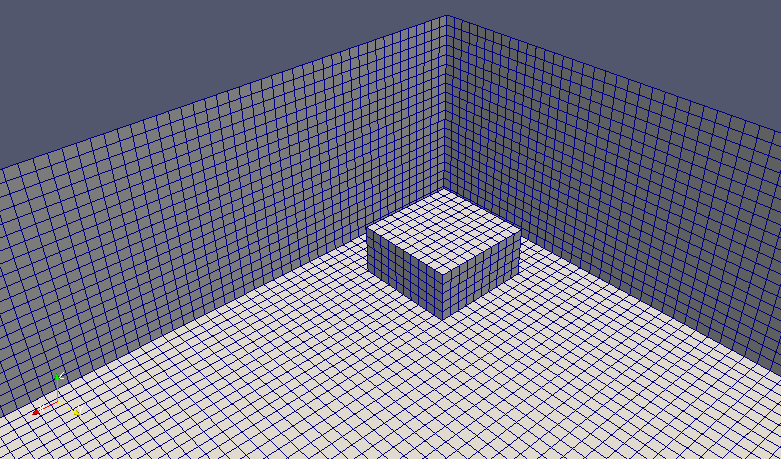Meshes (around the heating body)

The calculation result is as follows.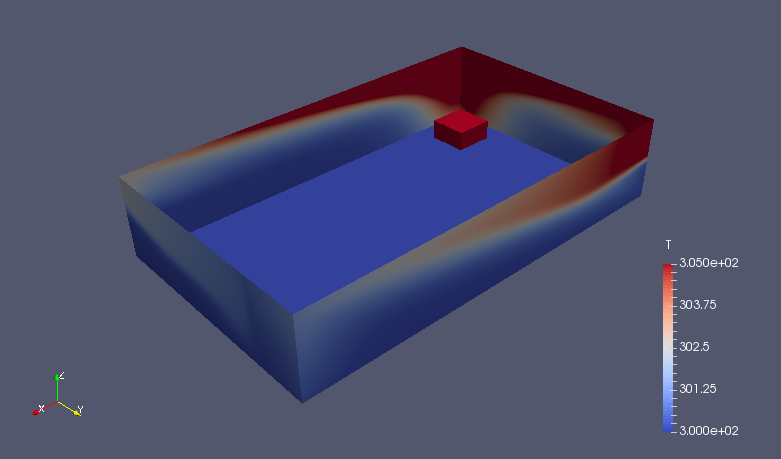Temperature (T)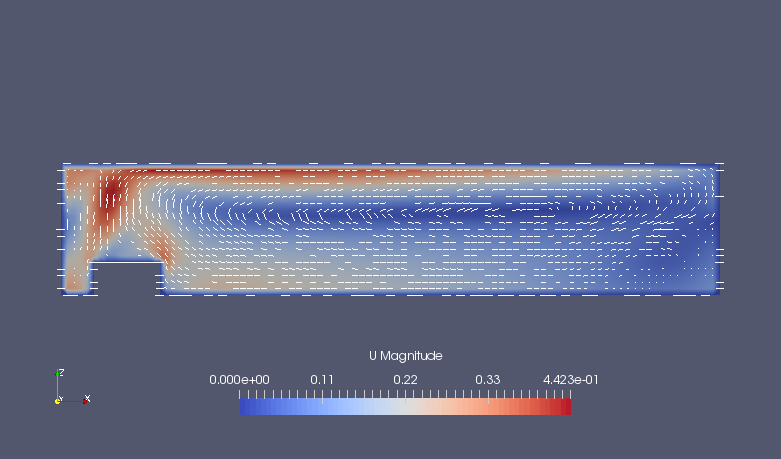Flow velocity on XZ-plane (Y=1) (U)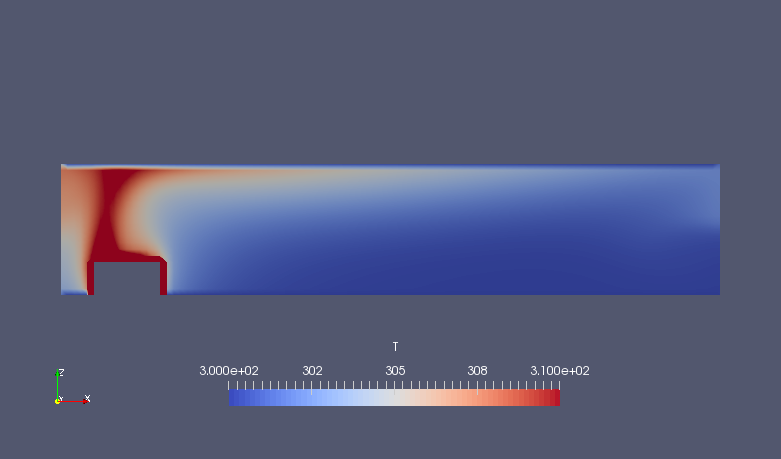Temperature on XZ-plane (Y=1) (T)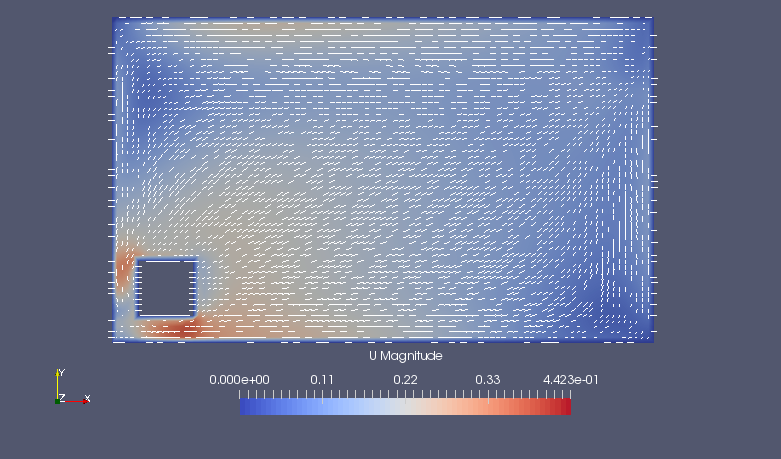Flow velocity on XY-plane (Z=0.25) (U)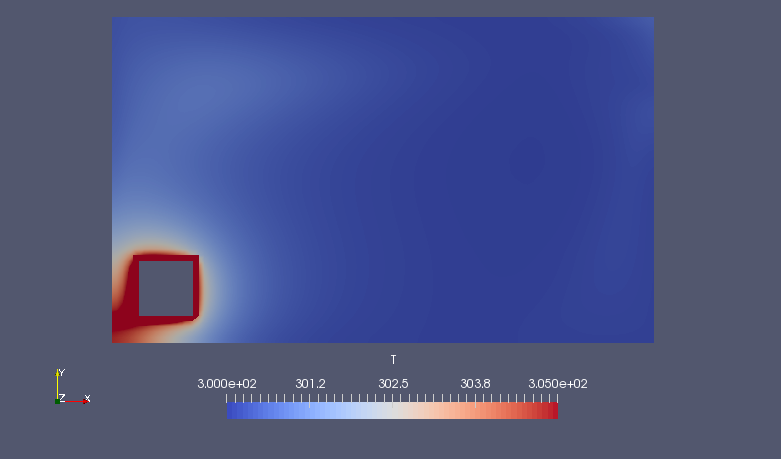Temperature on XY-plane (Z=0.25) (T)

The calculation results show that upward airflow occurs around the heating body and natural convection is generated.